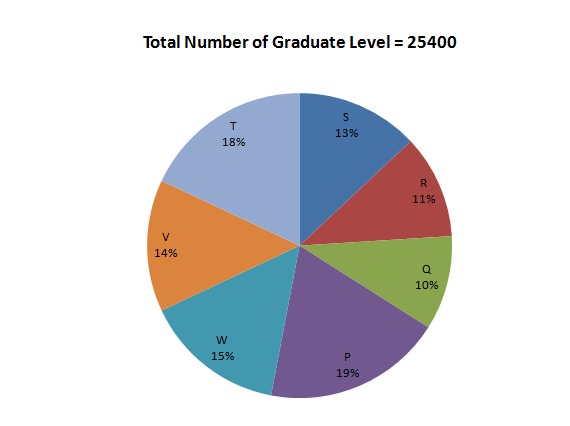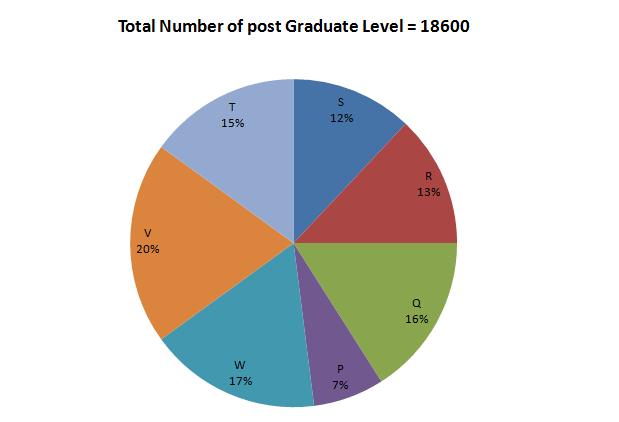Directions: The following pie-charts show the distribution of students of graduate and post-graduate levels in seven different institutes namely P,Q,R ,S, T,V and W.Question 1: How many students of institutes P and W are studying at graduate level?
(a) 9046
(b) 7486
(c) 8636
(d) 9256

Question 2: Total number of students studying at post-graduate level from institutes T and V is
(a) 6510
(b) 6070
(c) 6890
(d) 6230

Question 3: What is the total number of students studying at post-graduate and graduate levels in institute S?
(a) 5764
(b) 5534
(c) 5084
(d) 5246

Question 4: What is the nearest ratio between the number of students studying at post-graduate and graduate levels in institute R?

(a) 4 : 5
(b) 6 : 7
(c) 3 : 4
(d) 13 : 15

Question 5: What is the nearest ratio between the number of students studying at post-graduate level in institute V and graduate level in institute T?
(a) 6 : 7
(b) 8 : 9
(c) 4 : 5
(d) 3 : 4

Answer 1: (c) The total number of students studying in P and W in graduate level

= 19+15 = 34 % of the total students

Hence the required number = (34/100) x 25400  8636

The correct option is (c)

The total number of students studying in T and V in post graduate level

= 15+20 = 35 % of the total students.

Hence, the required number is (35/100) x 18600 = 6510

The, correct option is (a).

Total number of students studying in S

= 13% of 25400 + 12% of 18600 = 3302 + 2232 = 5534

The correct option is (b)

The number of students studying in R at post – graduate level is 13% of 18600

and at graduate level are 11% of 25400.

So, the required ratio = (13% of 18600)/(11% of 25400)

= (13 x 18600)/(11% of 25400) = (13 x 186)/(11 x 254)

= (13 x 186)/(11 x 254)

= (13 x 93)/11 x 127 = 0.86

Among the given choices, 13:15 is the answer (as it is equal to 0.86).

The number of students studying at post-graduate level in institute V = 20% of 18600 = 3720

number of students studying at graduate level in institute T = 18% of 25400 = 4572

So, the required ratio = 3720/4572 = 0.81

Among the given choices 4: 5 (which is equal to 0.8) is the closest to the given answer.

Thus, the correct option is (c).

### Want to explore more Data Interpretation Sets?

Get Posts Like This Sent to your Email
Updates for Free Live sessions and offers are sent on mail. Don't worry: we do not send too many emails..:)
Get Posts Like This Sent to your Email
Updates for Free Live sessions and offers are sent on mail. Don't worry: we do not send too many emails..:)